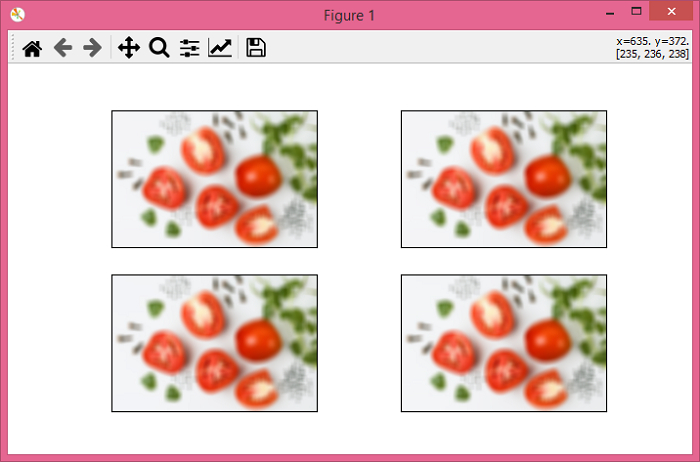# PyTorch – torchvision.transforms – GaussianBlur()

PyTorchServer Side ProgrammingProgramming

The torchvision.transforms module provides many important transformations that can be used to perform different types of manipulations on the image data. GaussianBlur() transformation is used to blur an image with randomly chosen Gaussian blur.

The GaussianBlur() transformation accepts both PIL and tensor images or a batch of tensor images. A tensor image is a PyTorch Tensor with shape [3, H, W], where H is the image height and W is the image width. A batch of tensor images is also a torch tensor with [B, 3, H, W] where B is the number of images in the batch.

## Syntax

torchvision.transforms.GaussianBlur(kernel_size, sigma=(0.1,.2))(img)
• kernel_size – Size of Gaussian kernel. It must be a list or tuple of two integers.

• sigma – Standard deviation used in creating the Gaussian kernel.

• img – PIL image or tensor image to be blurred.

It returns a Gaussian blurred image.

## Steps

We could use the following steps to blur an image with a randomly chosen Gaussian blur −

• Import the required libraries. In all the following examples, the required Python libraries are torch, Pillow, and torchvision. Make sure you have already installed them.

import torch
import torchvision
import torchvision.transforms as T
from PIL import Image
• Read the input image. The input image is a PIL image or a torch tensor.

img = Image.open('spice.jpg')
• Define a transform to blur the input image with randomly chosen Gaussian blur.

transform = T.GaussianBlur(kernel_size=(7, 13), sigma=(0.1, 0.2))
• Apply the above-defined transform on the input image to blur the input image.

blurred_img = transform(img)
• Show the blurred image.

blurred_img.show()

## Input Image

This image is used as the input file in all the following examples.## Example 1

This example demonstrates how you can blur an input image with a randomly chosen Gaussian blur.

# import required libraries
import torch
import torchvision.transforms as T
from PIL import Image

img = Image.open('spice.jpg')

# define the transform to blur image
transform = T.GaussianBlur(kernel_size=(7, 13), sigma=(9, 11))

# blur the input image using the above defined transform
img = transform(img)

# display the blurred image
img.show()

## Output

It will produce the following output −The above output is the blurred image of original input image.

## Example 2

Let's take another example −

import torch
import torchvision.transforms as T
from PIL import Image
import matplotlib.pyplot as plt

img = Image.open('spice.jpg')

# define a transform with kernel size and sigma
transform = T.GaussianBlur(kernel_size=(19, 23), sigma=(20, 25))

# apply the above transform on input image
blurred_imgs = [transform(img) for _ in range(4)]
fig = plt.figure(figsize=(7,4))
rows, cols = 2,2
for j in range(0, len(blurred_imgs)):
plt.imshow(blurred_imgs[j])
plt.xticks([])
plt.yticks([])
plt.show()

## Output

It will produce the following output −Each of the output image is blurred with a random Gaussian blur chosen from a given range.